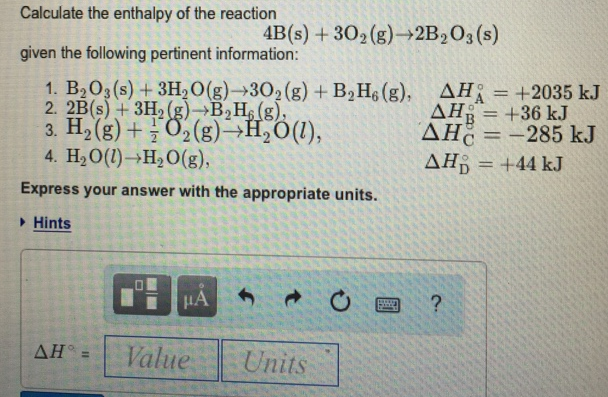# Problem: Calculate the enthalpy of the reaction 4B (s) + 3O2 (g) → 2B2O3 (s) given the following pertinent information: 1. B2O3 (s) + 3H2O (g)  → 3O2 (g) + B2H6 (g), ΔH°A = +2035 kJ 2. 2B (s) + 3H2 (g) → B2H6 (g), ΔH°B = +36kJ 3. H2 (g) + 1/2O2 (g) → H2O (l), ΔH°C = -285 kJ 4. H2O (l) → H2O (g), ΔH°D = +44 kJExpress your answer with the appropriate units.

🤓 Based on our data, we think this question is relevant for Professor Gonzalez's class at CSUF.

###### FREE Expert Solution###### Problem Details

Calculate the enthalpy of the reaction

4B (s) + 3O2 (g) → 2B2O3 (s) given the following pertinent information:

1. B2O3 (s) + 3H2O (g)  → 3O2 (g) + B2H6 (g), ΔH°A = +2035 kJ

2. 2B (s) + 3H2 (g) → B2H6 (g), ΔH°B = +36kJ

3. H2 (g) + 1/2O(g) → H2O (l), ΔH°= -285 kJ

4. H2O (l) → H2O (g), ΔH°D = +44 kJ